### Fake data, part 2: The black swan distribution

May 2017: This is from my other blog that's no longer online. The original comments are no longer available, but you are welcome to add more.

In the previous part when I wrote about the logistic distribution, I observed that it fit the S&P 500 daily returns pretty well out to 5 standard deviations (much better than the normal distribution) but broke down in the tails beyond.

That has been part of a weeks-long quest to find a distribution that fits, with the criterion that it must be closed-form; that is, we shouldn't need numerical methods to solve for the cumulative distribution or its inverse.

Why do this? Because I want to generate an endless stream of artificial market data for analysis. Not only do I want my artificial market to exhibit behavior that is statistically similar a real-world market, but I also want closed-form expressions for ease of programming into Excel or whatever I'll use for analysis. The normal distribution fails on both counts.

A normal distribution can't model real-world markets, with their many flat periods punctuated by "black swan" events — high-impact, unpredictable events beyond what one would normally expect. You need something with a taller peak and fatter tails. The logistic distribution gets a little bit closer, but not close enough. I need a black swan distribution!

There's a temptation to start with a probability density function of the desired shape (that fits the data) and go from there. It took me quite a while to realize that I can't start out this way, because I'm unlikely to get closed form expressions for related things like cumulative and inverse cumulative distributions. Why? Because to get the cumulative distribution, one must integrate the probability density function. And integration is hard, even for Wolfram Alpha, which uses Mathematica as its math engine. Even the ubiquitous normal distribution has no closed-form integral solution.

But differentiation is easy compared to integration. Sophisticated tools like Mathematica can almost always solve those. If one starts with a cumulative distribution function, one can use Mathematica to find its derivative, and also to solve for the inverse cumulative distribution.

The logistic distribution got us close, so we'll start there. Here's the cumulative distribution (cdf), written in terms of hyperbolic functions rather than exponentials (it's easier to read that way):

$$F(x;\mu,s)=\frac{1}{2} + \frac{1}{2} \tanh \left( \frac{x-\mu}{2s} \right) ~ \textrm{ using} ~ s = \frac{\sigma\sqrt{3}}{\pi}$$

As I mentioned in the earlier article, the logistic distribution has a variance σ2, although it doesn't really have a "standard deviation" as with the normal distribution. However, it is convenient to use that term, so I'll continue doing so as long as we have a distribution with a shape parameter (s) that can be defined in terms of σ.

To get the probability density function (pdf), simply take the derivative with respect to x, that is dF/dx.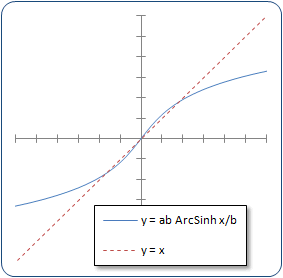We need to fatten the tails and sharpen the peak. One way is to substitute the argument to tanh with a nonlinear function of x such that f(x) ≈ x around the mean, but diverges further away from the mean. The inverse hyperbolic sine (ArcSinh or sinh−1) will do this. An example appears at the right. One can insert additional parameters to (a) adjust the steepness of the central area and (b) adjust the rate of divergence:

$$y=a b \sinh^{-1}\frac{x}{b}$$

After substituting this into the logistic pdf, extensive experiments determined that the parameter b = 1 works fine, although we'll leave it in for now. So our cdf becomes:

$$B(x;\mu,s)=\frac{1}{2} + \frac{1}{2} \tanh \left[a b \sinh^{-1}\left( \frac{x-\mu}{2 b s} \right)\right]\tag{1}$$

The B is for "black swan" to distinguish it from other distributions. The inverse cdf is: $$B^{-1}(p;\mu,s) = \mu - 2 b s \, \sinh \left[\frac {\tanh^{-1}(1-2p)} {a b} \right]\tag{2}$$ And the probability density function is the derivative of the cdf: $$\beta(x;\mu,s) = {\operatorname{d}F\over\operatorname{d}x} = \frac{a} {4s \cosh^2\left[a b \sinh^{-1}\left(\frac{x-\mu}{2 b s} \right ) \right ] \sqrt{\frac{(x-\mu)^2}{4 b^2 s^2}+1}}\tag{3}$$

It may look unwieldy, but it is closed form. The parameter a must be greater than 1; when a=1, the pdf reduces to something similar to a Cauchy distribution, which has undefined mean and variance.

We must attend to one final matter: the relationship between σ, s, and a. The relationship s = σ3/π doesn't work anymore. Unfortunately, we must get into numerical methods to solve for the relationship. Fortunately, we need to do it only once. The generalized relationship is

$$\frac{\sigma^2}{s^2} = \int_{-\infty}^\infty x^2 \beta(x)\,dx$$

where β(x) is our pdf above, setting μ=0 and s=1. We can use Solve My Math's definite integral solver, repeating over and over for different values of a. This integral solver returns a number, not a symbolic expression. Through experimentation and some help from an acquaintance who has Mathematica, I found a few discrete values of a that have symbolic expressions for σ2/s2 (when b=1):

$$\frac{\sigma^2}{s^2}= \begin{cases} (\frac{10}{\sqrt3}\, c-2 & a=6/5 \\ 3\sqrt{3 \over 2}\, c-2 & a=4/3 \\ \frac{8}{3}\,c-2 & a=3/2 \\ \sqrt{3}\,c-2 & a=2 \\ \frac{4}{3}\, c-2 & a=3 \\ \sqrt{3 \over2}\, c-2 &a=4 \\ \frac{\pi^2}{3a^2} & a \rightarrow \infty \end{cases} \quad \text{where} ~ c=\frac{\pi}{\sqrt 3}$$

Although it looks like there's a pattern, I could not find a closed-form expression that matched the curve. After days of investigation I hit upon the following approximation:

$$s \approx \frac{\sqrt{6}\,\sigma} {\pi \tanh^{-1} \left(\frac{1}{a}\right) \sqrt{\tanh^{-1} \left(\frac{1}{a}\right)^2+2}}\tag{4}$$

This approximation gives at least 3 significant digits of precision for a>1.4 and b=1. Good enough!

Now let's see how it fits. For the S&P 500 log daily returns the parameter a = 1.6 seems to work best, fitting the peak height, the peak width, and the tails of the distribution — not only for the whole data set from 1962 through 2009 but subsets as well. I tested it at 3-year intervals since 1962.

Look at these plots showing data from 1982 through 2009. The first plot uses a linear vertical scale to show how well it fits the central peak. The second plot uses a log vertical scale to show how well it fits the tails.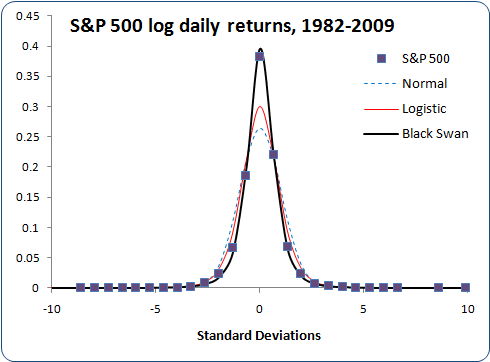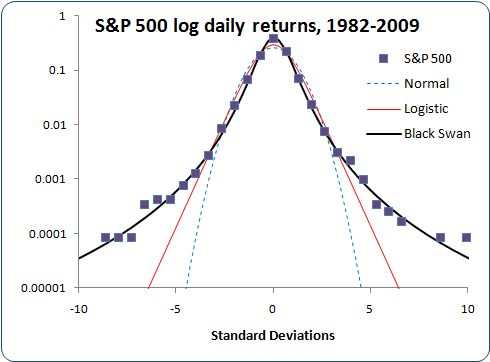Not bad at all! The black swan distribution fits the peak height, the peak width, and the tails (as seen in the log scale plot) better than anything else.

Now let's see how it works for other markets. In each case I use the mean and standard deviation of log returns to generate the distributions. I left the parameter a = 1.6 alone. It turned out that this value fit the tails well while fitting the narrowness of the peak at the same time. For smaller sample sizes, I noticed that a = 2.1 provides a somewhat better fit, although this value results in slightly thinner tails and a slightly lower peak. On the other hand, using a>2 may provide an advantage later in the form of finite kurtosis. For this black swan distribution, kurtosis goes infinite when a≤2. For now, I'll plot the distribution on other markets using a = 1.6.

Here's the distribution of 30-minute log returns on the EUR/USD FOREX market, again plotted in both linear and log vertical scales: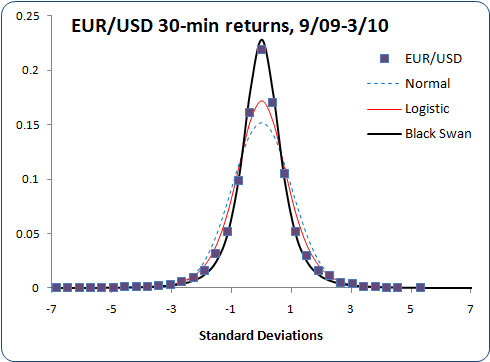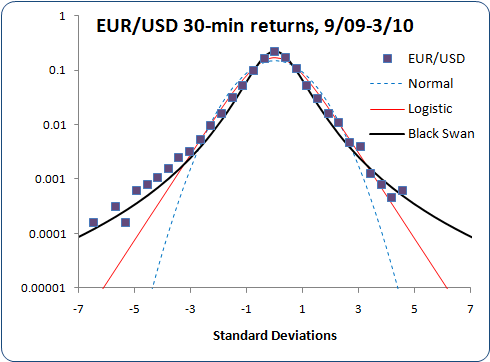Still pretty good!

Here's another, QQQQ daily returns, in linear and log scales: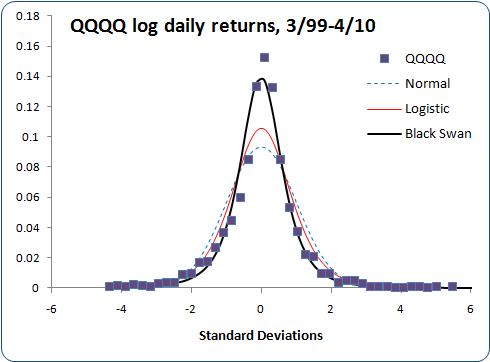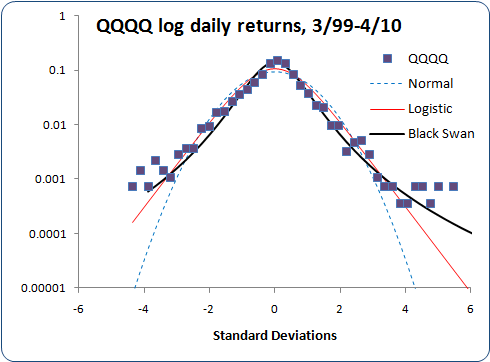The QQQQ histogram appears somewhat noisier because I have less than 3,000 daily prices, whereas I have over 6,000 for EUR/USD and over 12,000 for S&P 500. QQQQ also shows a bit of skew, which my distribution doesn't account for. Even so, it still fits better than either the normal or logistic distributions.

Let's look at something non-financial, say, spot prices for corn.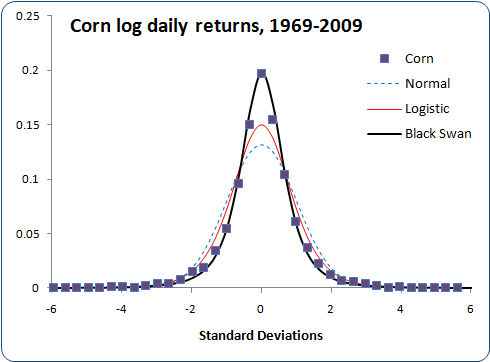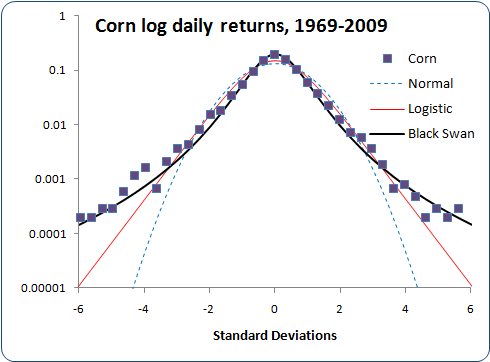Four unrelated markets, and my black swan distribution fits them all quite well, using the mean and standard deviation calculated from each market. I think I may have found something universal here. The fit is slightly better by setting b=1.02 (using a=1.6, s=2.19σ), but I couldn't work out a generalized approximation for b≠1. Using b=1 should be good enough.

Generating artificial market data is easy to do with the inverse cumulative distribution. Simply generate a probability p between 0 and 1, plug it into the inverse cdf equation above, and add the result to a running total. This generates a random walk with data distributed according to the black swan distribution.

But I have some more problems to resolve before I can generate a time series of artificial prices:

• I'd like to generate high, low, and close series, not just closes. To do this, I can generate, say, 1000 prices per "day" and use the high and low found. However, in the next part I will describe a problem encountered with this approach, and how I solve it.
• I need to determine if there are dependencies in direction or volatility from one close to the next in the real markets, and characterize those dependencies if they exist.

I'll tackle these problems in subsequent articles.